Related Articles
Descriptive Statistics in Julia
• Last Updated : 12 Oct, 2020

Julia is an appropriate programming language to perform data analysis. It has various built-in statistical functions and packages to support descriptive statistics. Descriptive Statistics helps in understanding the characteristics of the given data and to obtain a quick summary of it.

Packages required for performing Descriptive Statistics in Julia:

• Distributions.jl: It provides a large collection of probabilistic distributions and related functions such as sampling, moments, entropy, probability density, logarithm, maximum likelihood estimation, distribution composition, etc.
• StatsBase.jl: It provides basic support for statistics. It consists of various statistics-related functions, such as scalar statistics, high-order moment computation, counting, ranking, covariances, sampling, and empirical density estimation.
• CSV.jl: It is used reading and writing Comma Separated Values(CSV) files.
• Dataframes.jl: It is used for the creation of different data structures.
• StatsPlots.jl: It is used to represent various statistical plots.

Steps to perform Descriptive Statistics in Julia:

Step 1: Installing Required Packages

The following command can be used to install the required packages:

```Using Pkg

```

Step 2: Importing the Required Packages

## Julia

 `# Descriptive Statistics in Julia ` `# Importing required packages  ` `# to perform descriptive statistics ` ` `  `# For random variable creation ` `using Distributions  ` ` `  `# For basic statistical operations ` `using StatsBase ` ` `  `# For reading and writing CSV files ` `using CSV  ` ` `  `# For creation of Data Structures  ` `using DataFrames   ` ` `  `# For representing various plots ` `using StatsPlots`

Step 3: Creating stimulated Data (Random Variables)

Let’s create various variables with random data values

Example:

## Julia

 `# Descriptive Statistics in Julia ` `# Importing required packages  ` `# to perform descriptive statistics ` ` `  `# For random variable creation ` `using Distributions  ` ` `  `# For basic statistical operations ` `using StatsBase ` ` `  `# For reading and writing CSV files ` `using CSV  ` ` `  `# For creation of Data Structures  ` `using DataFrames   ` ` `  `# For representing various plots ` `using StatsPlots  ` ` `  `# Uniform Distribution ` `Age ``=` `rand(``10``:``95``, ``100``);   ` ` `  `# Weighted Uniform Distribution ` `BloodGrp ``=` `rand([``"A"``, ``"B"``, ``"O"``, ``"AB"``], ``100``);`

Step 4: Performing Descriptive statistics

The common statistical functions in Julia include mean(), median(), var(), and std() for calculating mean, median, variance and standard deviation of the data respectively. The more convenient functions aredescribe(), summarystats() from StatsBase package to perform descriptive statistics.

Example:

## Julia

 `# Descriptive Statistics in Julia ` `# Importing required packages  ` `# to perform descriptive statistics ` ` `  `# For random variable creation ` `using Distributions   ` ` `  `# For basic statistical operations ` `using StatsBase ` ` `  `# For reading and writing CSV files ` `using CSV   ` ` `  `# For creation of Data Structures   ` `using DataFrames   ` ` `  `# For representing various plots ` `using StatsPlots   ` ` `  `# Uniform Distribution ` `Age ``=` `rand(``10``:``95``, ``100``);   ` ` `  `# Weighted Uniform Distribution ` `BloodGrp ``=` `rand([``"A"``, ``"B"``, ``"O"``, ``"AB"``], ``100``);  ` ` `  `# mean of Age variable ` `mean(Age) ` ` `  `# median of Age variable ` `median(Age) ` ` `  `# Variance of Age variable ` `var(Age) ` ` `  `# Standard deviation of Age variable ` `std(Age) ` ` `  `# Descriptive statistics of Age variable ` `describe(Age) ` ` `  `# summarystats function excludes type ` `summarystats(Age)`

Output: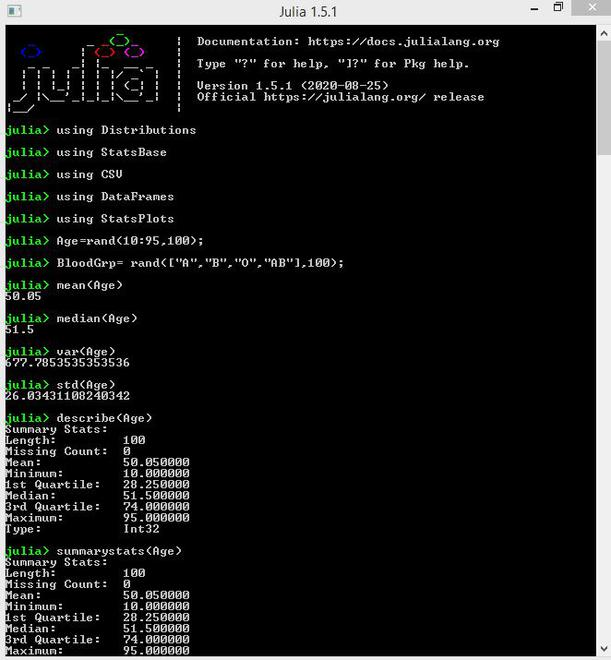Step 5: Creating data frames from the stimulated data

Stimulated data should be stored in data frame objects for performing manipulation operations easily.

Example:

## Julia

 `# Descriptive Statistics in Julia ` `# Importing required packages  ` `# to perform descriptive statistics ` ` `  `# For random variable creation ` `using Distributions   ` ` `  `# For basic statistical operations ` `using StatsBase ` ` `  `# For reading and writing CSV files ` `using CSV   ` ` `  `# For creation of Data Structures   ` `using DataFrames   ` ` `  `# For representing various plots ` `using StatsPlots   ` ` `  `# Uniform Distribution ` `Age ``=` `rand(``10``:``95``, ``100``);   ` ` `  `# Weighted Uniform Distribution ` `BloodGrp ``=` `rand([``"A"``, ``"B"``, ``"O"``, ``"AB"``], ``100``);   ` ` `  `# Creation of data frame ` `DF ``=` `DataFrame(AGE ``=` `Age, BGRP ``=` `BloodGrp); ` ` `  `# number of rows and columns ` `size(DF) ` ` `  `# First 5 rows ` `head(DF, ``5``) ` ` `  `# Last 5 rows ` `tail(DF, ``5``) ` ` `  `# Selecting specific data only ` `# Data in which BGRP=AB is printed ` `DFAB ``=` `DF[DF[:BGRP] .``=``=``"AB"``, :]  ` ` `  `# Data in which AGE>50 is printed ` `DF50 ``=` `DF[DF[:AGE] .>``90``, :]`

Output: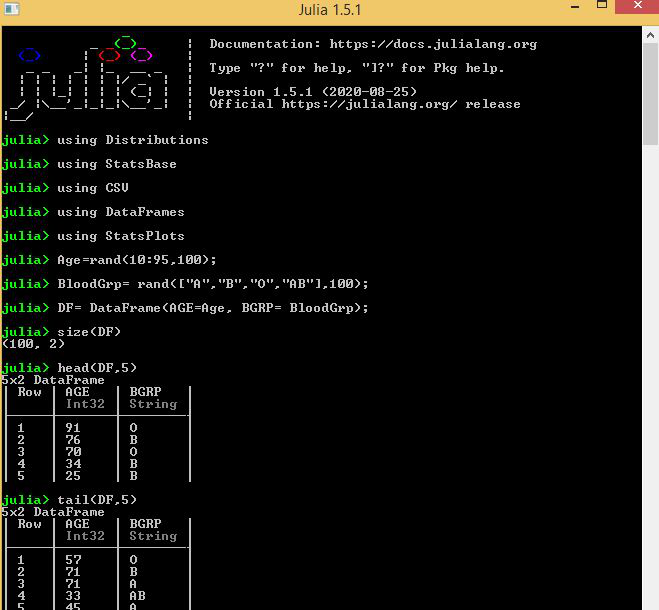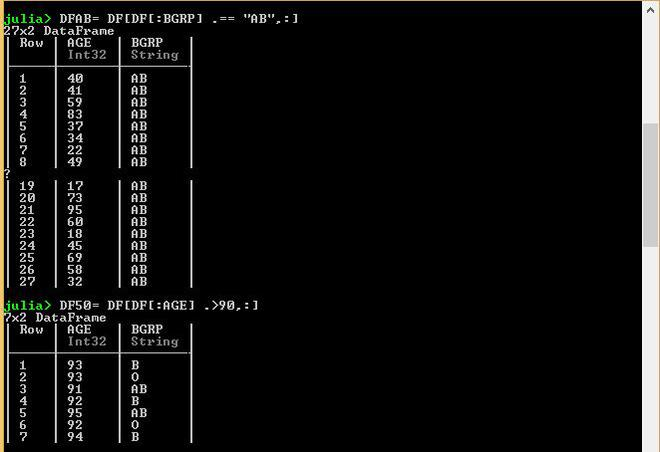Step 6: Descriptive Statistics using DataFrame Objects

• describe() function can be used to perform descriptive statistics of the data objects.

Example:

## Julia

 `# Descriptive Statistics in Julia ` `# Importing required packages  ` `# to perform descriptive statistics ` ` `  `# For random variable creation ` `using Distributions   ` ` `  `# For basic statistical operations ` `using StatsBase ` ` `  `# For reading and writing CSV files ` `using CSV   ` ` `  `# For creation of Data Structures   ` `using DataFrames   ` ` `  `# For representing various plots ` `using StatsPlots   ` ` `  `# Uniform Distribution ` `Age ``=` `rand(``10``:``95``, ``100``);   ` ` `  `# Weighted Uniform Distribution ` `BloodGrp ``=` `rand([``"A"``, ``"B"``, ``"O"``, ``"AB"``], ``100``);   ` ` `  `# Creation of data frame ` `DF ``=` `DataFrame(AGE ``=` `Age, BGRP ``=` `BloodGrp); ` ` `  `# Perform descriptive statistics of data frame ` `describe(DF)`

Output: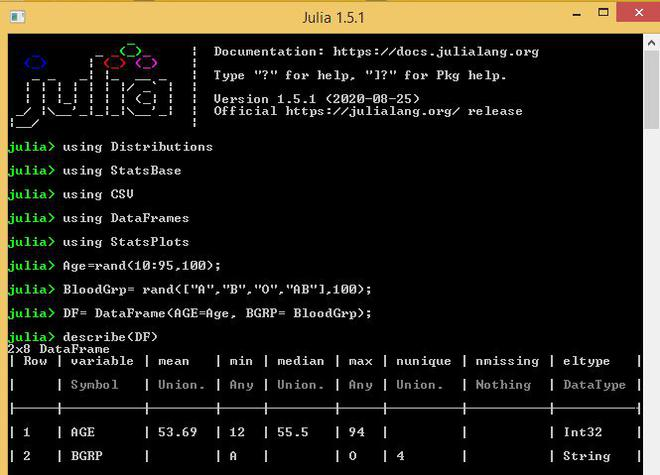• by() function is used to calculate the number of elements in the sample space of a categorical variable.

Example:

## Julia

 `# Descriptive Statistics in Julia ` `# Importing required packages  ` `#to perform descriptive statistics ` ` `  `# For random variable creation ` `using Distributions   ` ` `  `# For basic statistical operations ` `using StatsBase ` ` `  `# For reading and writing CSV files ` `using CSV   ` ` `  `# For creation of Data Structures   ` `using DataFrames   ` ` `  `# For representing various plots ` `using StatsPlots   ` ` `  `# Uniform Distribution ` `Age ``=` `rand(``10``:``95``, ``100``);   ` ` `  `# Weighted Uniform Distribution ` `BloodGrp ``=` `rand([``"A"``, ``"B"``, ``"O"``, ``"AB"``], ``100``);   ` ` `  `# Creation of data frame ` `DF ``=` `DataFrame(AGE ``=` `Age, BGRP ``=` `BloodGrp); ` ` `  `# Counting the number of rows  ` `# with blood groups A,B,O,AB ` `by(DF, :BGRP, DF``-``> DataFrame(Total ``=` `size(DF, ``1``))) ` ` `  `# Counting the number of rows ` `# with blood groups A, B, O, AB  ` `# using size argument ` `by(DF, :BGRP, size)`

Output: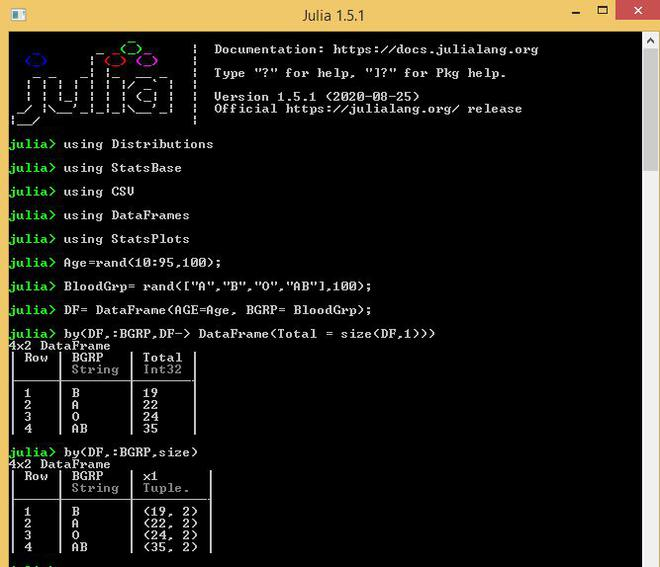• The descriptive statistics of different numerical variables can be calculated after separating them by categorical variables.

Example:

## Julia

 `# Descriptive Statistics in Julia ` `# Importing required packages  ` `# to perform descriptive statistics ` ` `  `# For random variable creation ` `using Distributions   ` ` `  `# For basic statistical operations ` `using StatsBase ` ` `  `# For reading and writing CSV files ` `using CSV   ` ` `  `# For creation of Data Structures   ` `using DataFrames   ` ` `  `# For representing various plots ` `using StatsPlots   ` ` `  `# Uniform Distribution ` `Age ``=` `rand(``10``:``95``, ``100``);   ` ` `  `# Weighted Uniform Distribution ` `BloodGrp ``=` `rand([``"A"``, ``"B"``, ``"O"``, ``"AB"``], ``100``);   ` ` `  `# Creation of data frame ` `DF ``=` `DataFrame(AGE ``=` `Age, BGRP ``=` `BloodGrp); ` ` `  `# Mean AGE of Blood groups A, B, AB, O ` `by(DF, :BGRP, DF``-``>mean(DF.AGE)) ` ` `  `# Using the describe function  ` `# we can get the complete descriptive statistics ` `by(DF, :BGRP, DF``-``>describe(DF.AGE))`

Output: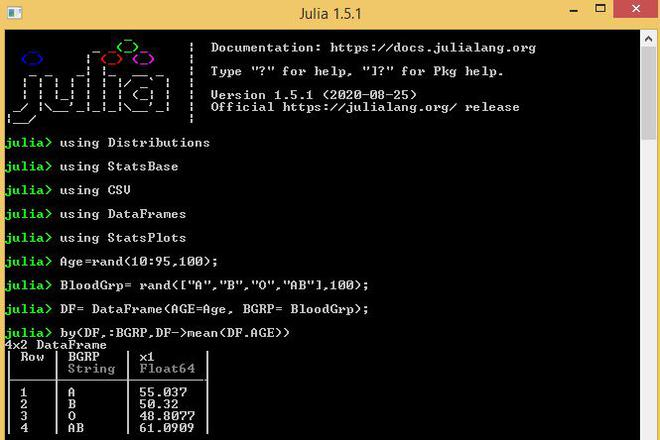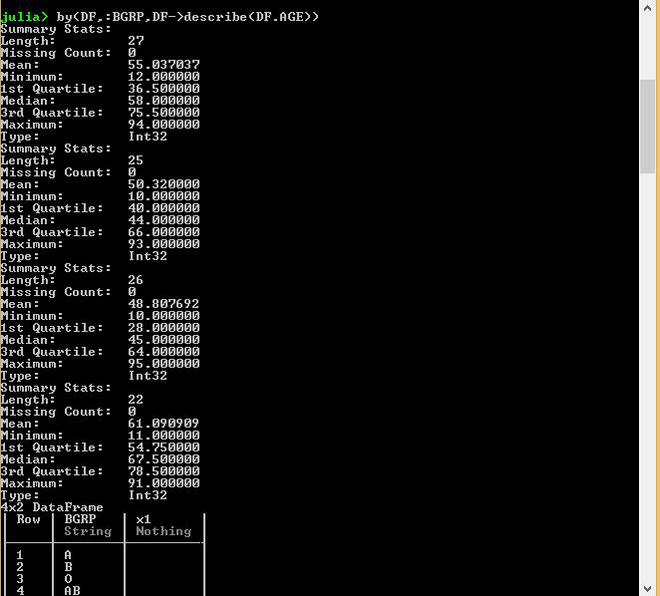Step 7: Visualizing Data using Plots

DataFrames package works well with the Plots package using the macro functions. In the following code:

• Let’s analyze the Age distribution of the Blood groups A, B, AB, O:

Example:

## Julia

 `# Descriptive Statistics in Julia ` `# Importing required packages  ` `# to perform descriptive statistics ` ` `  `# For random variable creation ` `using Distributions   ` ` `  `# For basic statistical operations ` `using StatsBase ` ` `  `# For reading and writing CSV files ` `using CSV   ` ` `  `# For creation of Data Structures   ` `using DataFrames   ` ` `  `# For representing various plots ` `using StatsPlots   ` ` `  `# Uniform Distribution ` `Age ``=` `rand(``10``:``95``, ``100``);   ` ` `  `# Weighted Uniform Distribution ` `BloodGrp ``=` `rand([``"A"``, ``"B"``, ``"O"``, ``"AB"``], ``100``);   ` ` `  `# Creation of data frame ` `DF ``=` `DataFrame(AGE ``=` `Age, BGRP ``=` `BloodGrp); ` ` `  `# Plotting density plot ` `@df` `DF density( ` `   ``:AGE, ` `   ``group ``=` `:BGRP, ` `   ``xlab ``=` `"Age"``, ` `   ``ylab ``=` `"Distribution"`     `)`

Output: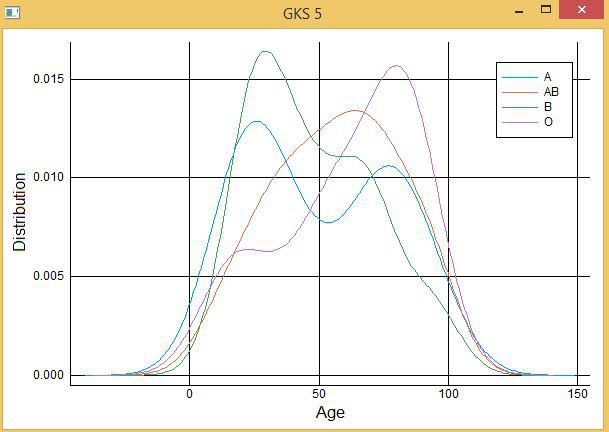• Let’s create a box-and-Whisker plot of Age :

Example:

## Julia

 `# Descriptive Statistics in Julia ` `# Importing required packages to perform descriptive statistics ` ` `  `# For random variable creation ` `using Distributions   ` ` `  `# For basic statistical operations ` `using StatsBase ` ` `  `# For reading and writing CSV files ` `using CSV   ` ` `  `# For creation of Data Structures   ` `using DataFrames   ` ` `  `# For representing various plots ` `using StatsPlots   ` ` `  `# Uniform Distribution ` `Age ``=` `rand(``10``:``95``, ``100``);   ` ` `  `# Weighted Uniform Distribution ` `BloodGrp ``=` `rand([``"A"``, ``"B"``, ``"O"``, ``"AB"``], ``100``);   ` ` `  `# Creation of data frame ` `DF ``=` `DataFrame(AGE ``=` `Age, BGRP ``=` `BloodGrp); ` ` `  `# Plotting Box plot ` `@df` `DF boxplot( ` `  ``:AGE, ` `  ``xlab ``=` `”Age”, ` `  ``ylab ``=` `”Distribution”     ` `)`

Output: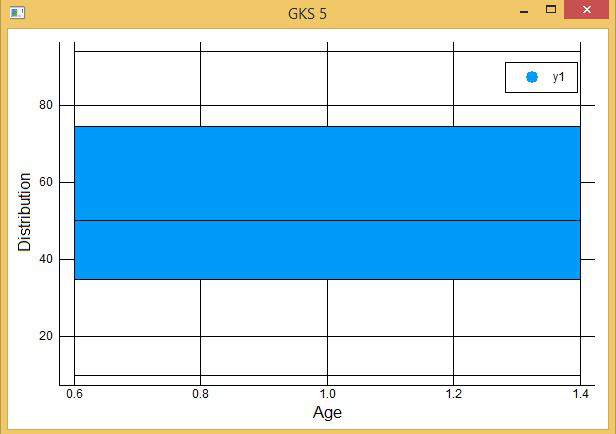My Personal Notes arrow_drop_up
Recommended Articles
Page :